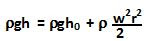## Sunday, June 28, 2009

### Irodov Problem 1.330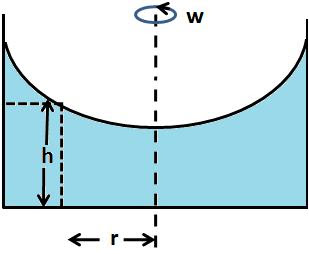Essentially the problem asks us to find the function h = f(r), i.e. how the height of the fluid depends on the distance from the axis of rotation.

Basically what happens is that as the fluid rotates, each section of the rotating fluid exerts a centrifugal force (as seen from the frame rotating with the axis) on the next section of the fluid. Consequently the next section of the fluid increases in height to generate enough hydrostatic pressure to compensate for the centrifugal force.

Let us first start by computing the hydrostatic force exerted by an infinitesimally thin section of rotating fluid of height h and width dr.At a depth x from the surface the hydrostatic pressure exerted by the fluid is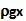. The force exerted over a thin section of height dx at a this depth x is given by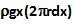. The net hydrostatic force exerted by the entire section of liquid is given by,The centrifugal force exerted by this section of liquid as it rotates is given by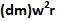. Here dm is the mass of the infinitesimally thin section of the liquid and is given by. The centrifugal force exerted by this section of the liquid is thus given by.Now let us consider too adjacent infinitesimally thin sections of the liquid of height h and h+dh.
The right (lower height column) exerts a hydrostatic force of F(h) to the left while the left (higher column) exerts hydrostatic force F(h+dh) to the right. The right column also exerts a centrifugal force oftowards the left. For equilibrium we have,As dh->0 and dr->0 we can neglect second order terms in (1) such as dh squared and obtain,Here h0 is the height of the fluid at the center of the cylinder. In practice knowing the total volume of the liquid in the container ho can be found.

b) The hydrostatic pressure at the bottom of the cylinder any radius r is simply